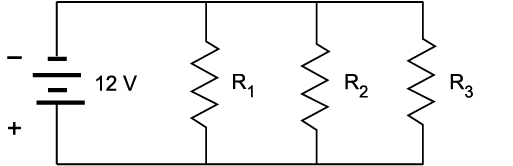# 3.2: Parallel Circuits

•• Contributed by Camosun College
The parallel circuit is probably the most common type of circuit you will encounter. Loads in power distribution systems are usually connected in parallel with each other in one way or another. A parallel circuit is constructed by connecting the terminals of all the individual load devices so that the same value of voltage appears across each component. In Figure $$\PageIndex{1}$$, you can see that each of the three resistors receives the same voltage from the source.Figure $$\PageIndex{1}$$: A parallel circuit (CC BY-NC-SA; BC Industry Training Authority)Figure $$\PageIndex{2}$$: Schematic of a parallel circuit (CC BY-NC-SA; BC Industry Training Authority)